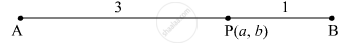Share

# Given A(4, –3), B(8, 5). Find the Coordinates of the Point that Divides Segment Ab in the Ratio 3 : 1. - Geometry

ConceptDivision of a Line Segment

#### Question

Given A(4, –3), B(8, 5). Find the coordinates of the point that divides segment AB in the ratio 3 : 1.

#### SolutionLet the coordinate of the point which divide the line AB in the ratio 3 : 1 be P(a, b)

$a = \frac{3 \times 8 + 1 \times 4}{3 + 1} = \frac{24 + 4}{4} = 7$

$b = \frac{3 \times 5 + 1 \times \left( - 3 \right)}{3 + 1} = \frac{15 - 3}{4} = 3$

P(a, b) = (7, 3)

Is there an error in this question or solution?

#### APPEARS IN

Balbharati Solution for Balbharati Class 10 Mathematics 2 Geometry (2018 to Current)
Chapter 5: Co-ordinate Geometry
Problem set 5 | Q: 17 | Page no. 123
Solution Given A(4, –3), B(8, 5). Find the Coordinates of the Point that Divides Segment Ab in the Ratio 3 : 1. Concept: Division of a Line Segment.
S